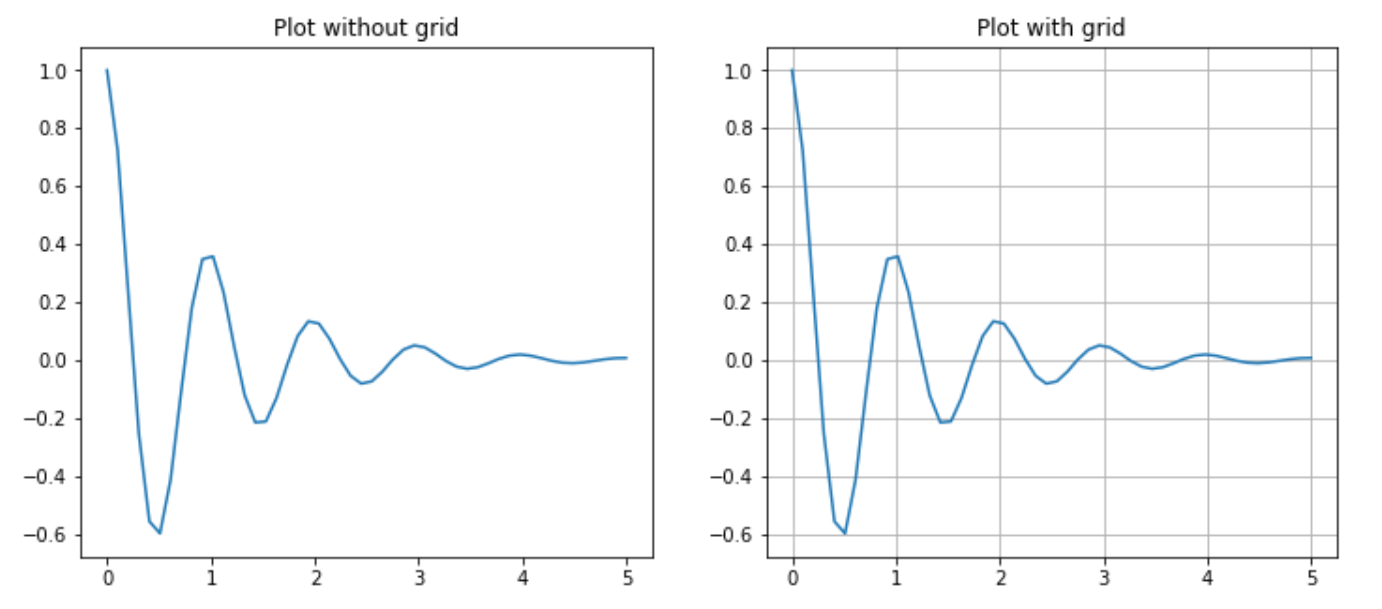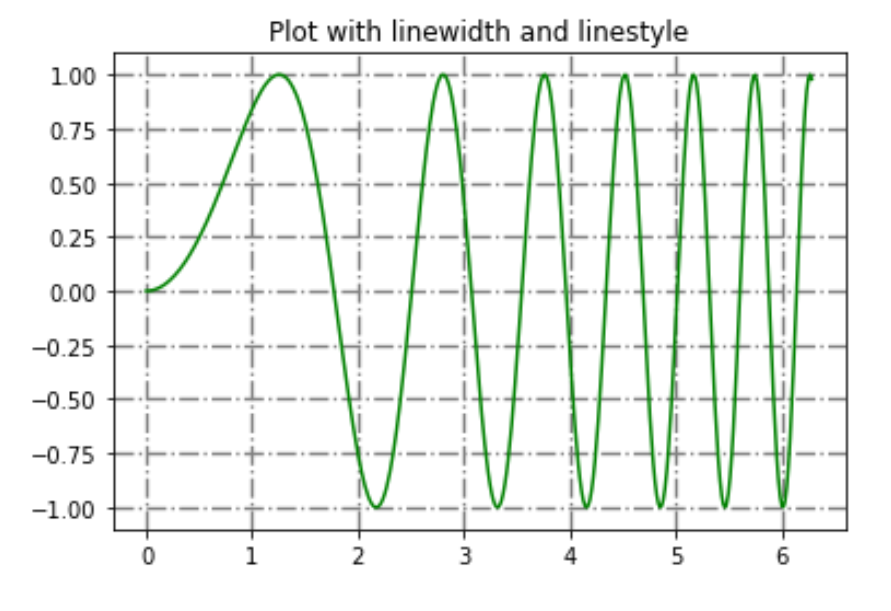Grids in Matplotlib

• Last Updated : 22 Apr, 2020

Grids are made up of intersecting straight (vertical, horizontal and angular) or curved lines used to structure our content. Matplotlib helps us to draw plain graphs but it sometimes necessary to use grids for better understanding and get a reference for our data points. Thus, Matplotlib provides a grid() for easy creation of gridlines with tonnes of customization.

matplotlib.pyplot.grid()

Syntax: matplotlib.pyplot.grid(b=None, which=’major’, axis=’both’, **kwargs)

Parameters:
b: bool value to specify whether to show grid-lines. Default is True
which: The grid lines to apply changes. Values: {‘major’, ‘minor’, ‘both’}
axis: The axis to apply changes on. Values: {‘both’, ‘x’, ‘y’}
**kwargs: Optional line properties

Returns: This function doesn’t return anything.

The grid() sets the visibility of grids by specifying a boolean value (True/False). We can also choose to display minor or major ticks or both. Also, color, linewidth and linestyle can be changed as additional parameters. Let’s look at some examples:

Example #1:

 # Implementation of matplotlib functionimport matplotlib.pyplot as pltimport numpy as np  # dummy datax1 = np.linspace(0.0, 5.0)y1 = np.cos(2 * np.pi * x1) * np.exp(-x1)  # creates two subplotsfig, (ax1, ax2) = plt.subplots(1, 2, figsize = (12, 5))  # Plot without gridax1.plot(x1, y1)ax1.set_title('Plot without grid')  # plot with gridax2.plot(x1, y1)ax2.set_title("Plot with grid")  # draw gridlinesax2.grid(True)  plt.show()

Output:Example #2:
Now let’s draw gridlines using extra line properties such as color, linestyle and linewidth.

 # Implementation of matplotlib functionimport matplotlib.pyplot as pltimport numpy as np  # dummy datax = np.linspace(0, 2 * np.pi, 400)y = np.sin(x ** 2)  # set graph colorplt.plot(x, y, 'green')  # to set titleplt.title("Plot with linewidth and linestyle")  # draws gridlines of grey color using given # linewidth and linestyleplt.grid(True, color = "grey", linewidth = "1.4", linestyle = "-.")  plt.show()

Output:My Personal Notes arrow_drop_up